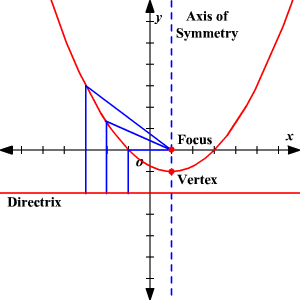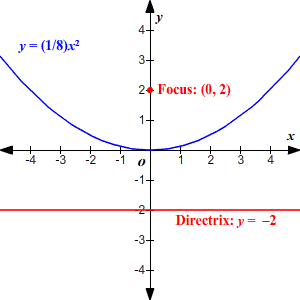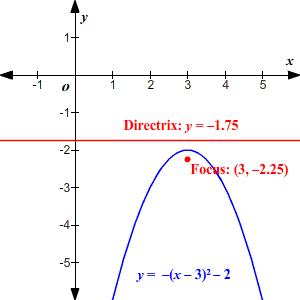# Focus of a Parabola

A parabola is set of all points in a plane which are an equal distance away from a given point and given line. The point is called the focus of the parabola and the line is called the directrix.

The focus lies on the axis of symmetry of the parabola.### Finding the focus of a parabola given its equation

If you have the equation of a parabola in vertex form $y=a{\left(x-h\right)}^{2}+k$, then the vertex is at $\left(h,k\right)$ and the focus is $\left(h,k+\frac{1}{4a}\right)$.

Notice that here we are working with a parabola with a vertical axis of symmetry, so the $x$-coordinate of the focus is the same as the $x$-coordinate of the vertex.

Example 1:

Find the focus of the parabola $y=\frac{1}{8}{x}^{2}$.

Here $h=0$ and $k=0$, so the vertex is at the origin. The coordinates of the focus are $\left(h,k+\frac{1}{4a}\right)$ or $\left(0,0+\frac{1}{4a}\right)$.

Since $a=\frac{1}{8}$, we have

$\frac{1}{4a}=\frac{1}{\left(\frac{1}{2}\right)}$

$=2$

The focus is at $\left(0,2\right)$.Example 2:

Find the focus of the parabola $y=-{\left(x-3\right)}^{2}-2$.

Here $h=3$ and $k=-2$, so the vertex is at $\left(3,-2\right)$. The coordinates of the focus are $\left(h,k+\frac{1}{4a}\right)$ or $\left(3,-2+\frac{1}{4a}\right)$.

Here $a=-1$, so

$-2+\frac{1}{4a}=-2-\frac{1}{4}$

$=-2.25$

The focus is at $\left(3,-2.25\right)$.